Next: Levenshtein Distance Up: Sequence comparison Previous: Sequence comparison

# Basic Problem

We are interested in the notion of resemblance or similarity between two words x and y of length m and n respectively. A dual notion is to look at the distance between these two words.

A word x = x0x1···xm-1 of length m is a sequence of letters from an alphabet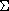. Thus, for 0 <= i <= m-1,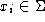.

The empty word denoted byhas a null length: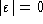.

A function d is a distance between words if:

•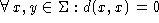and d(x,y) > 0 for all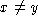;
•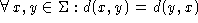;
• triangular inequality: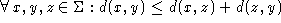.

Several distances between words can be considered:

• the prefix distance: dpref(x,y) = |x| + |y| - 2*lcp(x,y) where lcp(x,y) is the length of the longest common prefix of x and y;
• the suffix distance is defined in a similar way as the prefix distance;
• id. for the substring distance;
• the Hamming distance.

We are interested in a distance which enables to transform x into y using three kinds of basic operations: the substitution of a letter of x by a letter of y, the deletion of a letter of x or the insertion of a letter of y. A cost is associated to each of these operations and for each letter of the alphabet:

• Sub(a,b) is the cost of the substitution of the letter a by the letter b;
• Del(a) is the cost of the deletion of the letter a;
• Ins(b) is the cost of the insertion of the letter b.

The general problem consists of finding a sequence of such basic operations to transform x into y minimizing the total cost of the operations used. The total cost is equal to the sum of the costs of each of the basic operations. This cost is a distance on the words if Sub is a distance on the letters.

We are trying to minimize the distance between x and y which is generally the same (but not always) than maximizing the similarity between these two words.

The solution is not necessarily unique.

A solution can be given as a sequence of basic operations of substitutions, deletions and insertions. It can also be given in a similar way by an alignment.

For two words x of length m and y of length n such as m <= n. An alignment denoted by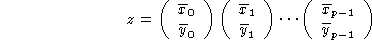or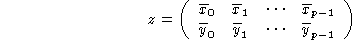of length p is such that:

• n <= p <= n+m;
•or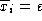for 0 <= i <= p-1 and 0 <= j <= m-1;
•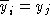or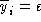for 0 <= i <= p-1 and 0 <= j <= n-1;
•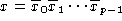;
•;
• for 0 <= i <= p-1,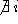such that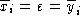.

An aligned pair of the type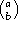with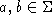indicates the substitution of the letter a by the letter b.
An aligned pair of the type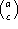with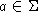indicates the deletion of the letter a.
An aligned pair of the type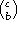with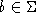indicates the insertion of the letter b.

In an alignement or in an aligned pair, the symbolis often replaced by the symbol -.

This problem can be easily stated in terms of graph. Let G = (V,E) be a labelled and weighted graph with an application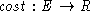which associates a cost to each edge of E and an application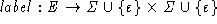which associates an aligned pair to each edge of E. The graph G is defined as follows:

• V is the set of vertices defined as follows: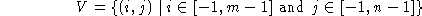• E is the set of edges defined as follows:
•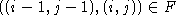for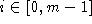and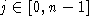and cost((i-1,j-1),(i,j)) = Sub(xi,yj) and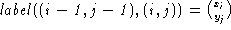;
•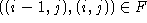forand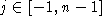and cost((i-1,j),(i,j)) = Del(xi) and;
•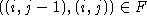for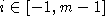andand cost((i,j-1),(i,j)) = Ins(yj) and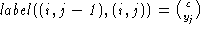.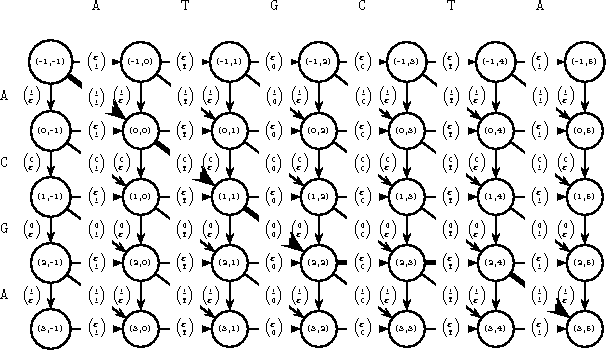Figure 1.1: Edit graph for x = ACGA and y = ATGCTA.

All the paths from (-1,-1) to (3,5) correspond to a different alignment between x and y. The thick edges correspond to the following alignment: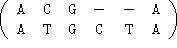.

It is only necessary to find a shortest path from the vertex (-1,-1) to the vertex (m-1,n-1). The less the cost the less distant are the two words x and y. As the graph G is acyclic it is possible to find a shortest path considering one time and only one each vertex. One had just to consider them in a topological order. Such an order can be obtained by considering the vertices row by row and from left to right within each row. Dynamic programming () can solve this problem.

Let T be a two-dimensional table with m+1 rows and n+1 columns. The value of each square T[i,j], for -1 <= i <= m-1 and -1 <= j <= n-1 depends only on the three squares T[i-1,j], T[i,j-1] and T[i-1,j-1].

Then for 0 <= i <= m-1 and 0 <= j <= n-1, T[i,j] is the minimum cost of a path from (-1,-1) to (i,j). The algorithm DYNAMIC-PROGRAMMING computes all the values of the table T.The algorithm DYNAMIC-PROGRAMMING clearly has a O(mn) time complexity.

The algorithm DYNAMIC-PROGRAMMING only computes the cost of the transformation of x into y. To get a sequence of basic operations to transform x into y or the corresponding alignment one has to trace back in the table T from square T[m-1,n-1] to square T[-1,-1] taking each time the right edge (see algorithm ONE-ALIGNMENT).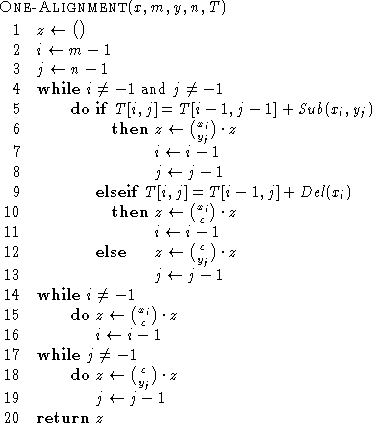If all the optimal alignments are required then a call to ALL-ALIGNMENT(x, m-1,y,n-1,,T) will produced them.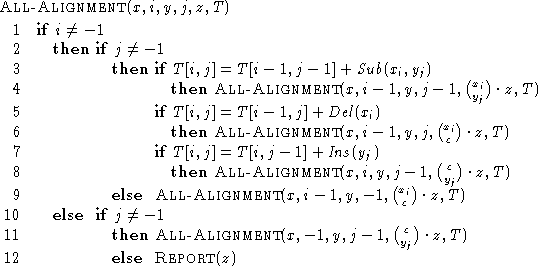In this case it is necessary to store all the values of the table T then this problem can be solve in O(mn) space complexity.

When only the cost of the transformation of x into y is needed it is easy to see that a space in O(min(m,n)) is sufficient since the computation of a row (respectively a column) only needs the values of the previous row (respectively column).

Furthermore it is possible to compute an alignment in linear space using a ``divide and conquer'' method (,  and ).Next: Levenshtein Distance Up: Sequence comparison Previous: Sequence comparison
e-mails: {Christian.Charras, Thierry.Lecroq}@laposte.net
Thu Feb 19 10:23:29 WET 1998